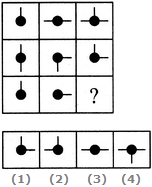# Non Verbal Reasoning - Figure Matrix - Discussion

Discussion Forum : Figure Matrix - Section 1 (Q.No. 3)
Directions to Solve

In each of the following questions, find out which of the answer figures (1), (2), (3) and (4) completes the figure matrix ?

3.

Select a suitable figure from the four alternatives that would complete the figure matrix.1
2
3
4
Explanation:
In each row, the third figure comprises of a black circle and only those line segments which are not common to the first and the second figures.
Discussion:
54 comments Page 1 of 6.

Rashmi said:   1 year ago
Yes, the given explanation is not valid because of a figure same as the second row last figure.
(5)

Sashi_karma said:   2 years ago
In each row,

After combining the 1st and 2nd figures, exclude the common line segments and copy the rest.

In the 1st row, there is not any common line segments. So all the line segments are combined.

In the 2nd row, south faced line segment is common. So, to exclude the south faced common line segment and copy the rest.

In the 3rd row, after combining the two figures, there is no common lines to exclude, so copy the rest.
(15)

Mohsin Attar said:   2 years ago
In a simple way it is the addition of lines.

1st+2nd=3rd.

Note: if the two lines from the 1st and 2nd box get collapses then the line gets substracted in 3rd box (i.e. In the 2nd row).

Ranjit chhetri said:   2 years ago
In the first row, in the first and second figure, the line segment is not overlapping thus the additional line is formed likewise in the second figure there is an overlapping between the line segments thus one line facing the bottom is removed. In a similar way in third figure there is no overlapping of the line segment thus it is added hence option is A.

Debraj Roy said:   2 years ago
The given explanation is correct. Assume there are 4 direction around the circle i.e; North, South, East and West.

Now, In the first row, the 1st circle has only the North direction and the 2nd circle has two directions i.e, East and West. So, basically, these two have no common direction. Thus the 3rd circle will have the direction which are Not Common in 1st and 2nd circles. i.e, North, East & West.

Similarly, In the 2nd row direction or the line segments which are not common in the 1st and 2nd circle are North and East.

Therefore, In the third row, the 3rd circle will have North & East direction or line segments because there are no common direction in the 1st amd 2nd circle to be omitted.

Debraj Roy said:   2 years ago
The given explanation is correct. Assume there are 4 direction around the circle i.e; North, South, East and West.

Now, In the first row, the 1st circle has only the North direction and the 2nd circle has two directions i.e, East and West. So, basically, these two have no common direction. Thus the 3rd circle will have the direction which are Not Common in 1st and 2nd circles. i.e, North, East & West.

Similarly, In the 2nd row direction or the line segments which are not common in the 1st and 2nd circle are North and East.

Therefore, In the third row, the 3rd circle will have North & East direction or line segments because there are no common direction in the 1st amd 2nd circle to be omitted.

Abhishek said:   2 years ago
@All.

The first column is the answer of the common of 2nd and 3rd column,

So in the last row which figures you will use to make the common match with the first column figure.

Kapil said:   3 years ago
Thank you @Mariaelena.

Sourish said:   4 years ago
@Selina.